# PATTERN RECOGNITION APPROACHES

Pattern Recognition Approaches | Pattern Recognition Tutorial | Minigranth

# Pattern Recognition Approaches : Introduction

• There are two fundamental pattern recognition approaches for implementation of pattern recognition system. These are:
• Statistical Pattern Recognition Approaches.
• Structural Pattern Recognition Approaches.

### Statistical Patter Recognition Approach

• Statistical Pattern Recognition Approach is in which results can be drawn out from established concepts in statistical decision theory in order to discriminate among data based upon quantitative features of the data from different groups. For example: Mean, Standard Deviation.
• The comparison of quantitative features is done among multiple groups.
• The various statistical approaches used are: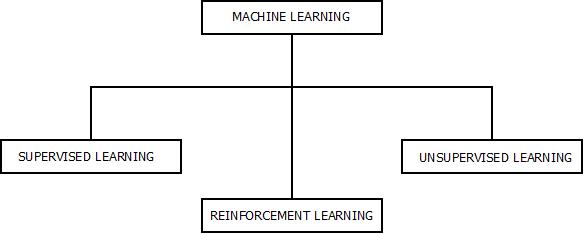Statistical Pattern Recognition Approaches

1. #### Bayesian Decision Theory

• Bayesian decision theory is a statistical model which is based upon the mathematical foundation for decision making.
• It involves probabilistic approach to generate decisions in order to minimize the complexity and risk while making the decisions.
• In Bayesian decision theory, it is assumed that all the respective probabilities are known because the decision problem can be viewed in terms of probabilities.
• It can be said that, Bayesian decision theory is dependent upon the Baye’s rule and posterior probability needs to be calculated in order to make decisions with the knowledge of prior probability. It can be calculated as :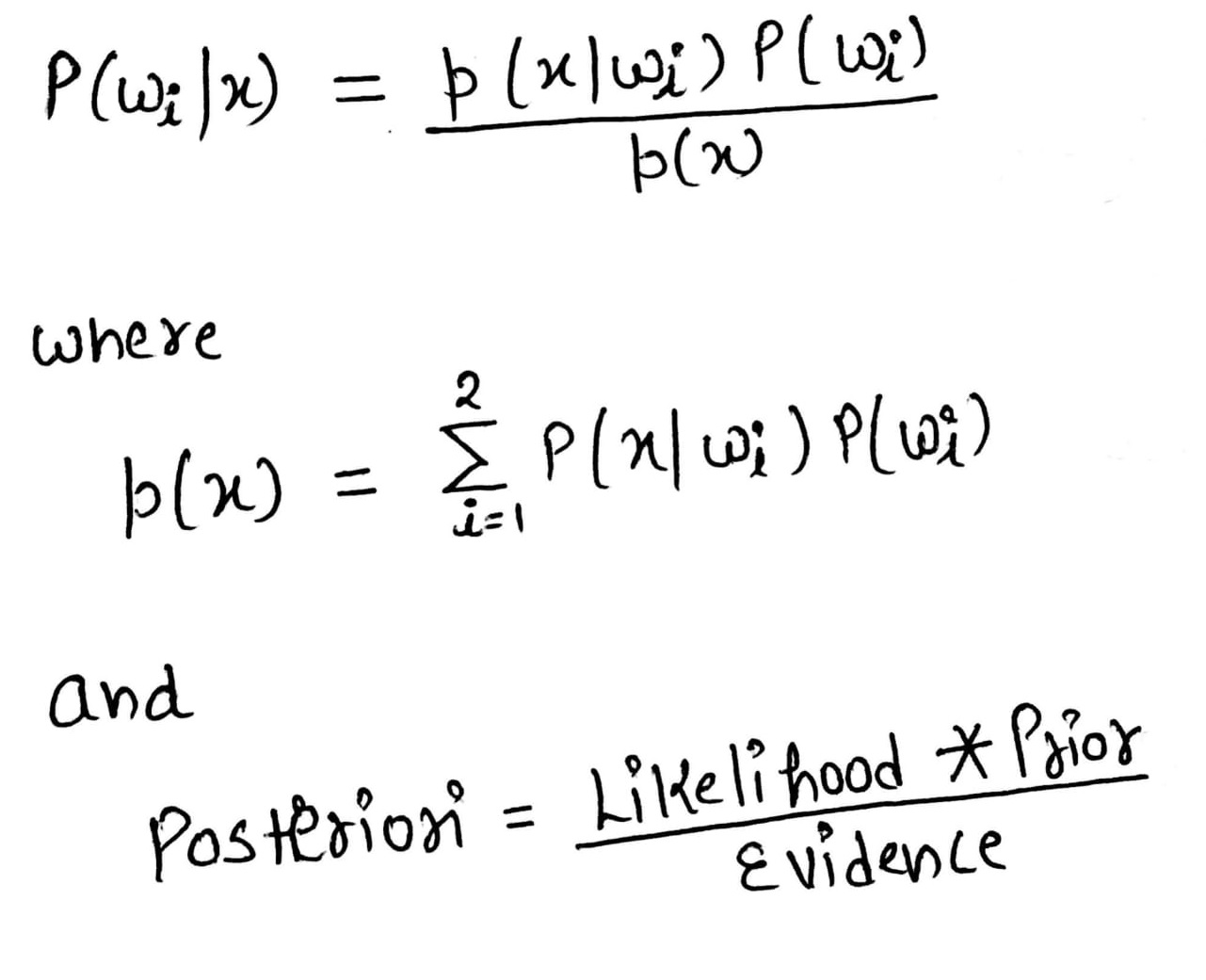Bayesian decision theory

• The difference is, Bayesian decision theory is the generalized form and can be used by replacing the scalar ‘‘x’’ with the feature vector “X”.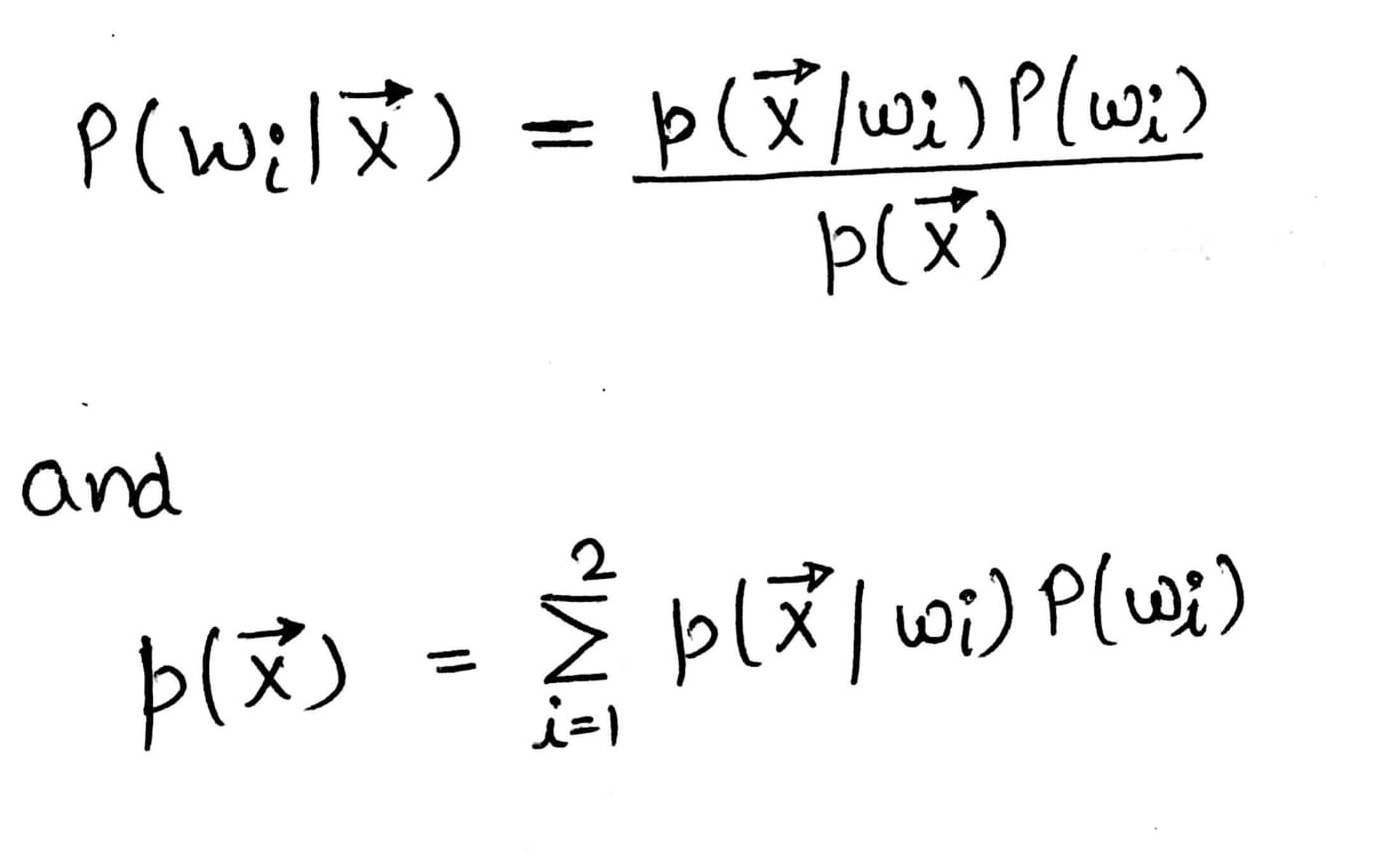Feature Vector : Bayesian Decision Theory

2. #### Normal Density

• Normal density curve is a bell shaped curve which is the most commonly used probability density function.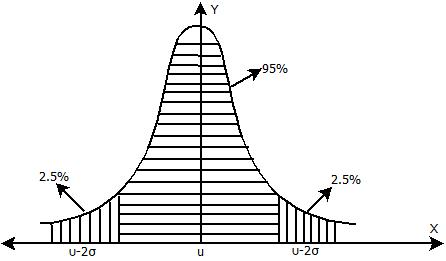Normal Density Curve : Pattern Recognition Approaches

• Since it is based upon the central limit theorem, normal density concept is able to handle larger number of cases.
• The Central Limit Theorem States that - “A given sufficiently large sample size from a population with a finite level of variance, the mean of all samples from the same population will be equal to mean of population”.
• The normal density function can be given by: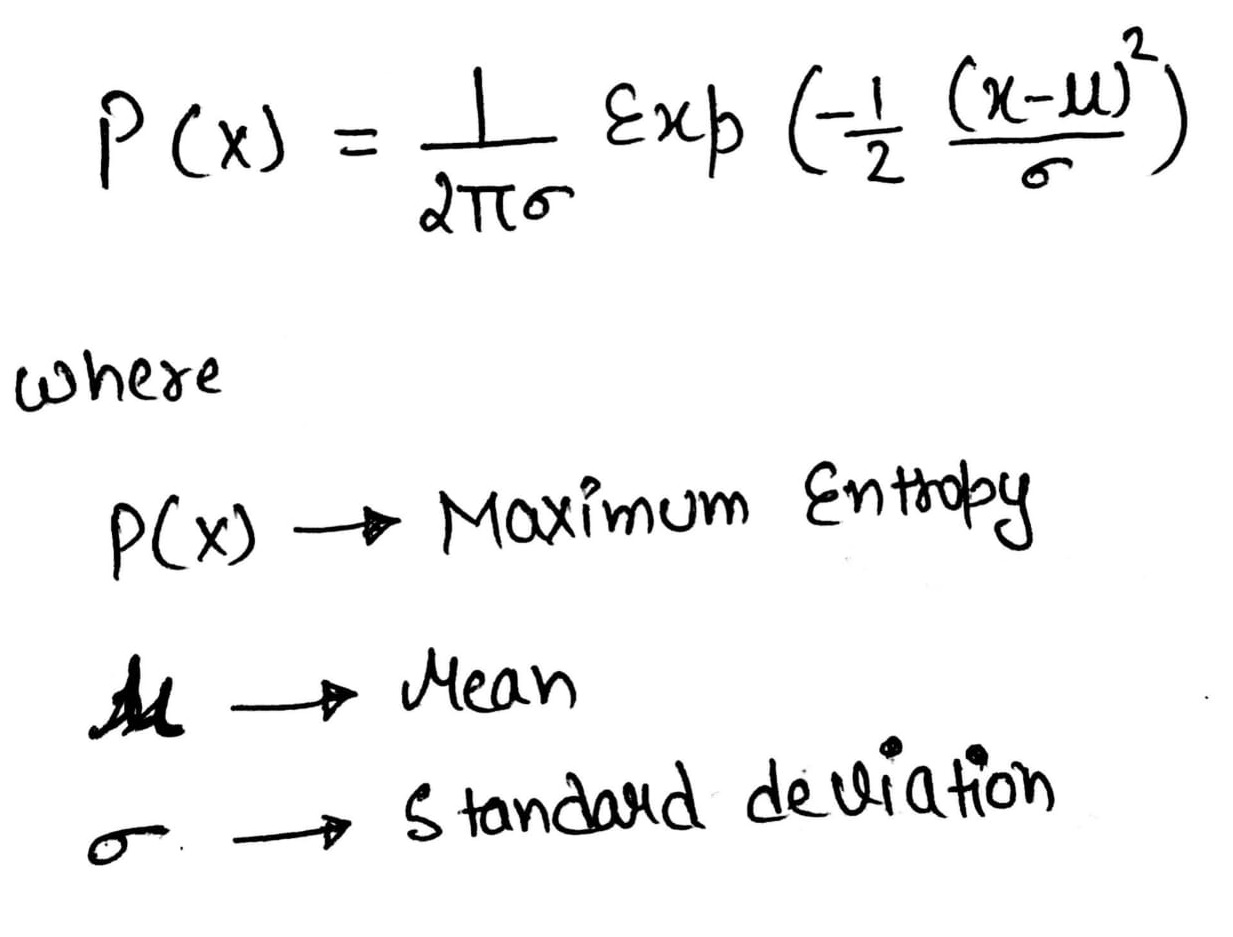Formula: Normal Density Function

3. #### Discriminant Function

• Pattern Classifiers can be represented with the help of discriminant functions.
• Discriminant Functions are used to check, which continuous variable discriminates between two or more naturally occurring groups.

### Structural Pattern Recognition Approach

• A Structural Approach is in which results can be drawn out from established concepts in structural decision theory in order to check interrelations and interconnections between objects inside single data sample.
• Sub-Patterns and relations are the structural features while applying an structural approach.
• For example : Graphs.